# 不容错过的Pandas小技巧：万能转格式、轻松合并、压缩数据，让数据分析更高效...## Pandas实用技巧

Pandas 在这一点上其实十分友好，只需添加一行代码。

DataFrame 转 HTML

import numpy as np
import pandas as pd
import random

n = 10
df = pd.DataFrame(
{
"col1": np.random.random_sample(n),
"col2": np.random.random_sample(n),
"col3": [[random.randint(0, 10) for _ in range(random.randint(3, 5))] for _ in range(n)],
}
)



df_html = df.to_html()
with open(‘analysis.html’, ‘w’) as f: f.write(df_html)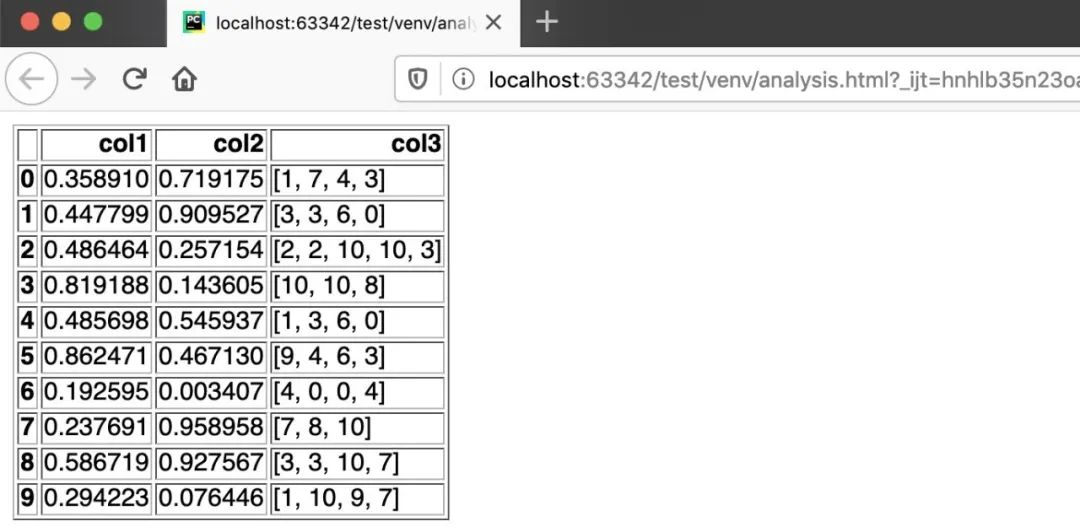DataFrame 转 LaTeX

df.to_latex()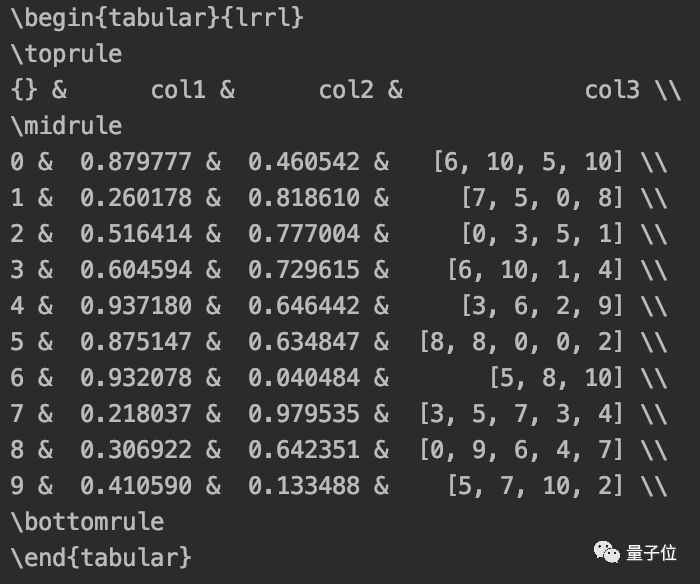DataFrame 转 Markdown

Pandas 同样为你考虑到了这一点：

print(df.to_markdown())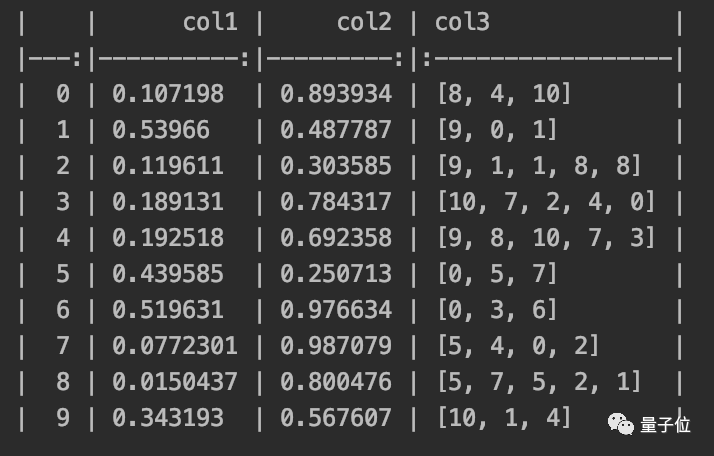DataFrame 转 Excel

df.to_excel(‘analysis.xlsx’)



DataFrame 转字符串

df.to_string()



## 5个鲜为人知的Pandas技巧

1、data_range

Pandas 的 data_range 覆盖了这一需求。

import pandas as pd
date_from = “2019-01-01”
date_to = “2019-01-12”
date_range = pd.date_range(date_from, date_to, freq=”D”)
print(date_range)


freq = “D”/“M”/“Y”，该函数就会分别返回按天、月、年递增的日期。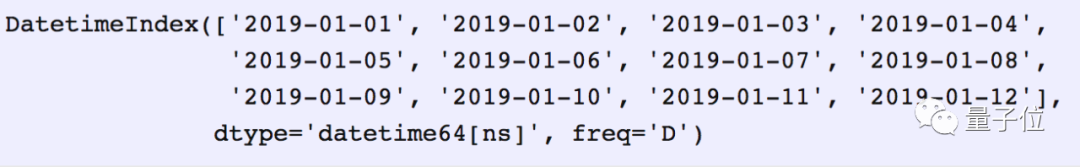2、合并数据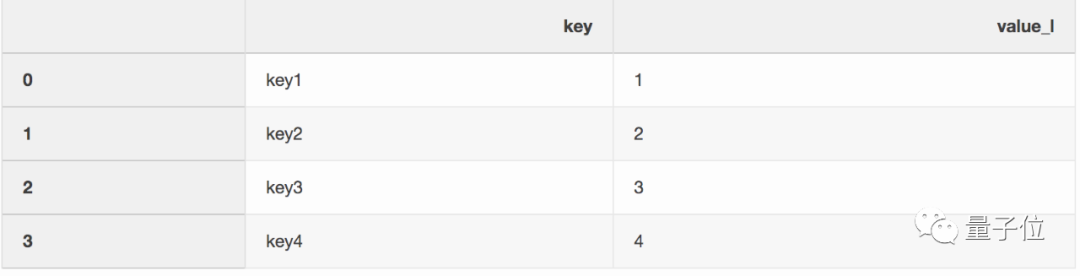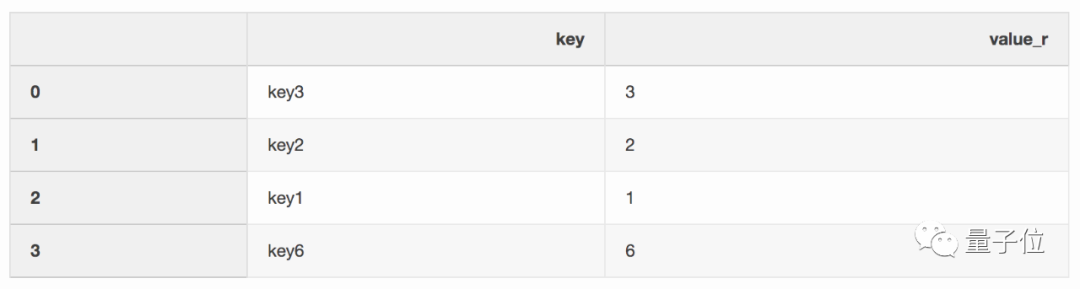df_merge = left.merge(right, on = ‘key’, how = ‘left’, indicator = True)



3、最近合并（Nearest merge）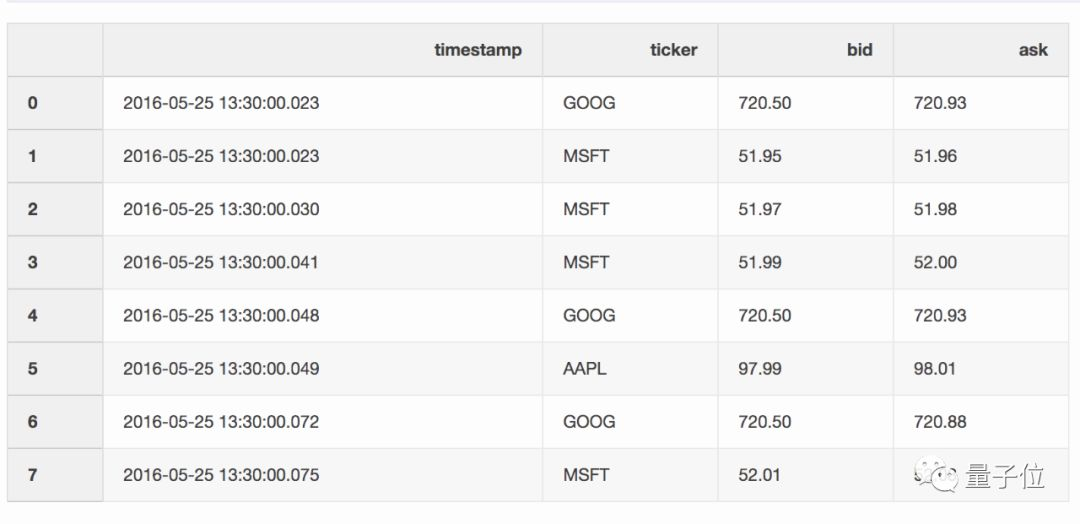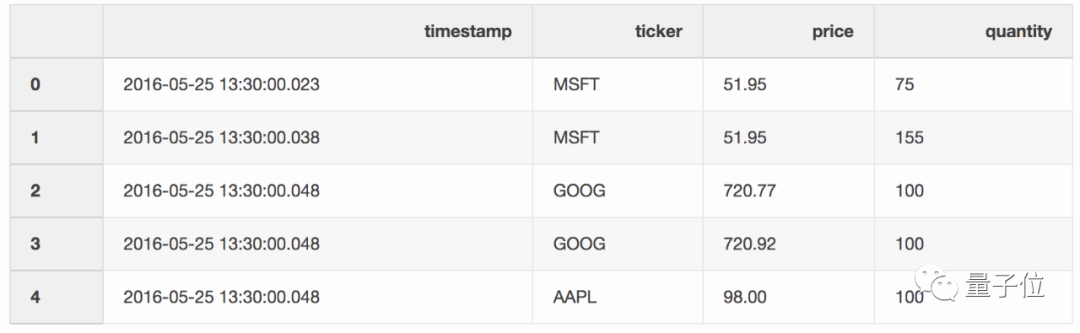pd.merge_asof(trades, quotes, on=”timestamp”, by=’ticker’, tolerance=pd.Timedelta(‘10ms’), direction=‘backward’)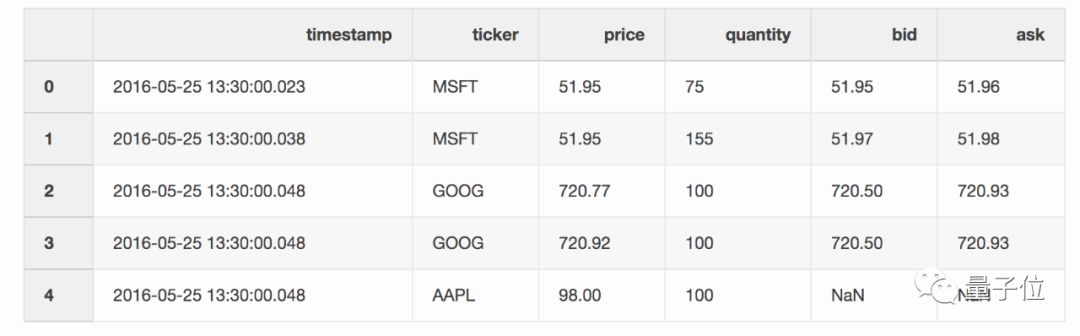4、创建Excel报告

import numpy as np
import pandas as pd

df = pd.DataFrame(np.array([[1, 2, 3], [4, 5, 6], [7, 8, 9]]), columns=["a", "b", "c"])

report_name =  example_report.xlsx
sheet_name =  Sheet1
writer = pd.ExcelWriter(report_name, engine= xlsxwriter )
df.to_excel(writer, sheet_name=sheet_name, index=False)



# define the workbook
workbook = writer.book
worksheet = writer.sheets[sheet_name]
# create a chart line object
chart = workbook.add_chart({ type :  line })
# configure the series of the chart from the spreadsheet
# using a list of values instead of category/value formulas:
#     [sheetname, first_row, first_col, last_row, last_col]
categories : [sheet_name, 1, 0, 3, 0],
values :     [sheet_name, 1, 1, 3, 1],
})
# configure the chart axes
chart.set_x_axis({ name :  Index ,  position_axis :  on_tick })
chart.set_y_axis({ name :  Value ,  major_gridlines : { visible : False}})
# place the chart on the worksheet
worksheet.insert_chart( E2 , chart)
# output the excel file
writer.save()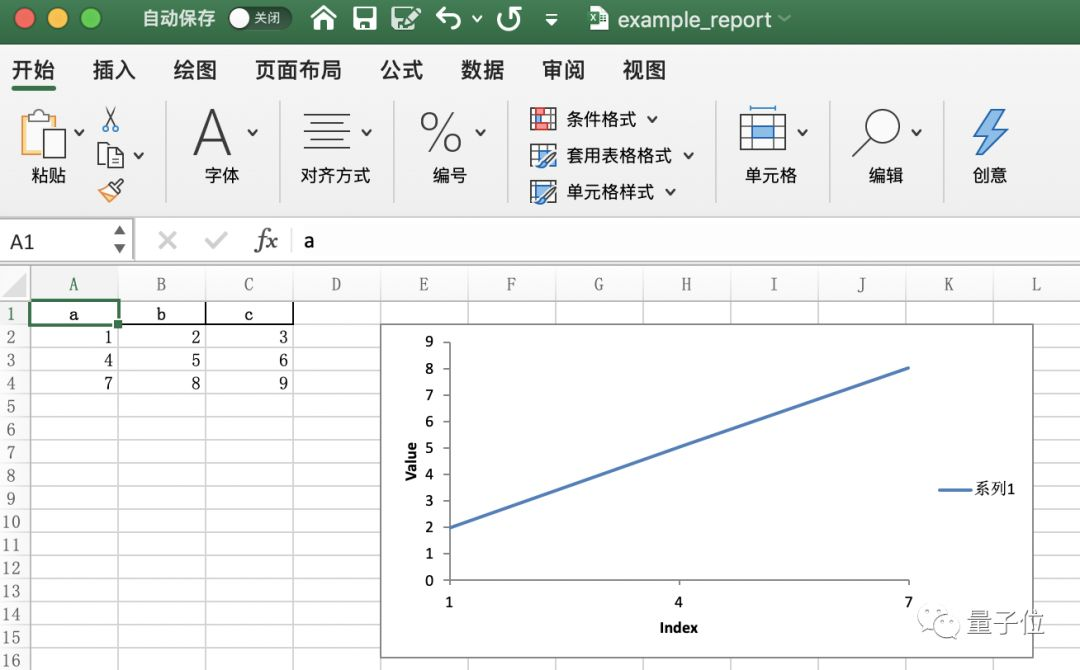5、节省磁盘空间

Pandas在保存数据集时，可以对其进行压缩，其后以压缩格式进行读取。

df = pd.DataFrame(pd.np.random.randn(50000,300))
df.to_csv(‘random_data.csv’, index=False)



df.to_csv(‘random_data.gz’, compression=’gzip’, index=False)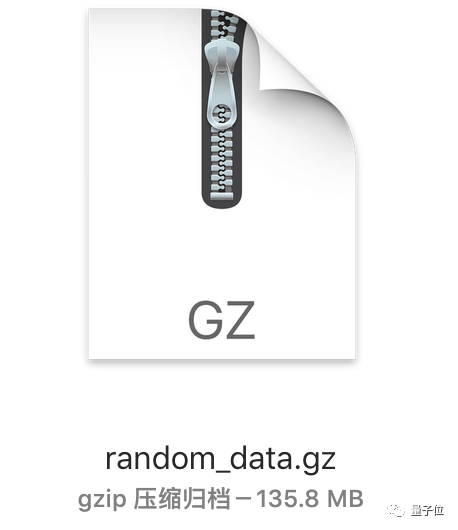gzip压缩文件可以直接读取：

df = pd.read_csv(‘random_data.gz’)



Talk is cheap, show me the code。学会了，就用起来吧

零基础学 Python(送价值109的视频课)，来这里
只需7天时间，跨进Python编程大门，已有3800+加入

【基础】0基础入门python，24小时有人快速解答问题；
【提高】40多个项目实战，老手可以从真实场景中学习python；
【直播】不定期直播项目案例讲解，手把手教你如何分析项目；
【分享】优质python学习资料分享，让你在最短时间获得有价值的学习资源；圈友优质资料或学习分享，会不时给予赞赏支持，希望每个优质圈友既能赚回加入费用，也能快速成长，并享受分享与帮助他人的乐趣。
【人脉】收获一群志同道合的朋友，并且都是python从业者
【价格】本着布道思想，只需 69元 加入一个能保证学习效果的良心圈子。
【赠予】价值109元 0基础入门在线课程，免费送给圈友们，供巩固和系统化复习©️2019 CSDN 皮肤主题: 大白 设计师: CSDN官方博客